# Quick Sorting Quick Selecting DivideandConquer Sorting Algorithm with

• Slides: 16Quick Sorting & Quick Selecting • Divide-and-Conquer Sorting Algorithm with Time-Complexing of O(n log n) • Algorithm for finding the Kth smallest element in unsorted array at O(n)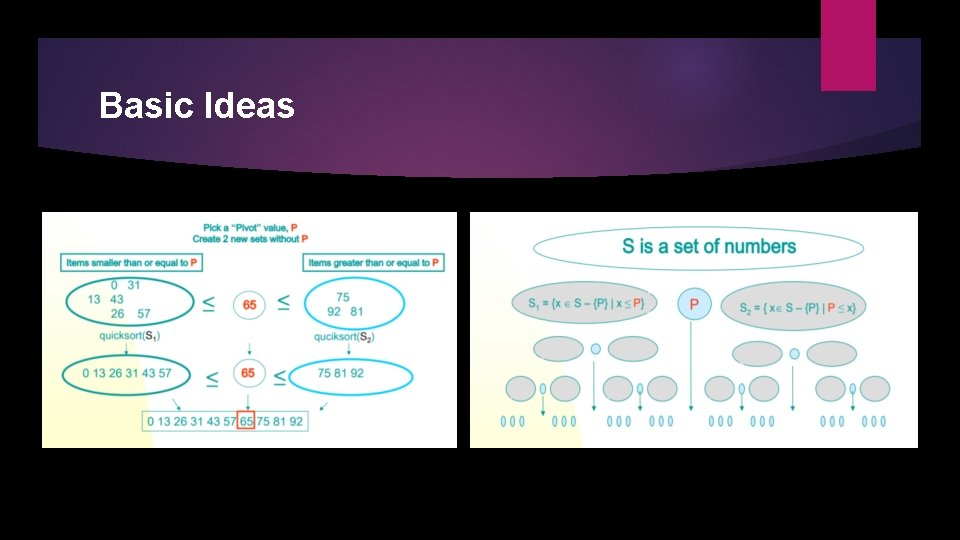Basic IdeasBasic Ideas Pick an element, say P (the pivot) Re-arrange the elements into 3 sub-blocks, 1. L: those less than or equal to ( ≤) P (the left-block S 1) 2. P: the pivot (the only element in the middle-block) 3. G: those greater than or equal to ( ≥) P (the right block S 2 ) Repeat the process recursively for the left- and right- sub-blocks. Return {quicksort( S 1), P, quicksort( S 2) }.Basic Ideas Note: The main idea is to find the “right” position for the pivot element P. After each “pass”, the pivot element, P, should be “in place”. Eventually, the elements are sorted since each pass puts at least one element (i. e. , P) into its final position. Issues: How to choose the pivot P ? How to partition the block into sub-blocks?Example of Partitioning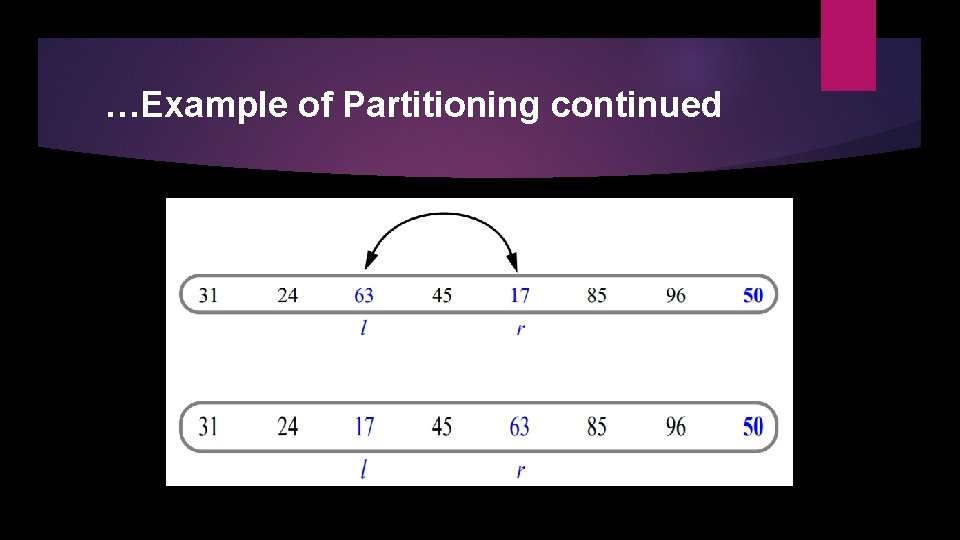…Example of Partitioning continued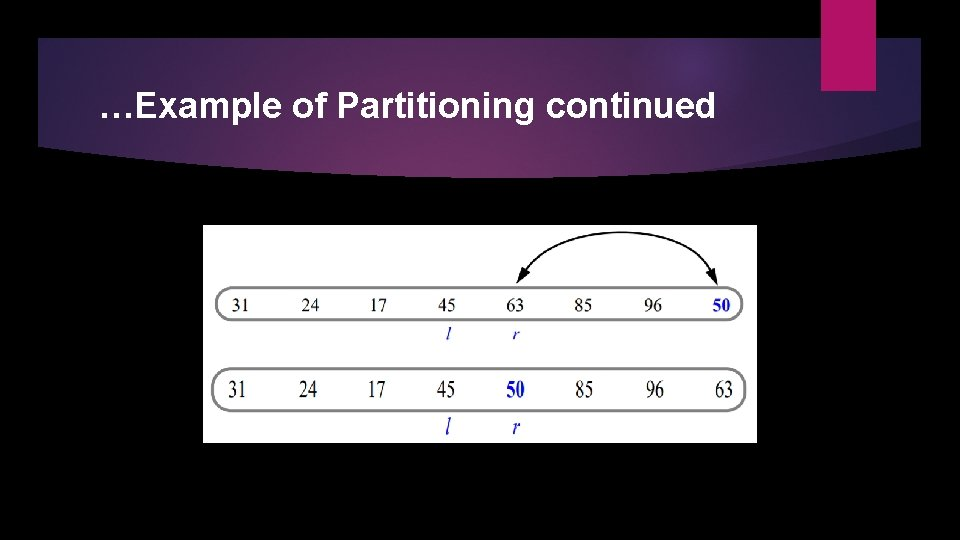…Example of Partitioning continuedAnother Quick Example… Another Quick Example continuedImplementation : Quick Sort function // low --> Starting index // high --> Ending index void quicksort (arr[], low, high) { if (low < high) { // pi is partitioning index // arr[pi] is now at right place pi = partition(arr, low, high); quick. Sort(arr, low, pi - 1); // Before pi quick. Sort(arr, pi + 1, high); // After pi } }Implementation : Partitioning function // This function sorts the array into left sub-block, pivot, right sub-block // and returns pivot index int partition (int arr[], int low, int high) { int pivot = arr[high]; // pivot int i = (low - 1); // Index of smaller element for (int j = low; j <= high-1; j++) { if (arr[j] <= pivot) { // If current element is smaller than or equal to pivot i++; // increment index of smaller element swap(&arr[i], &arr[j]); } } swap(&arr[i + 1], &arr[high]); return (i + 1); }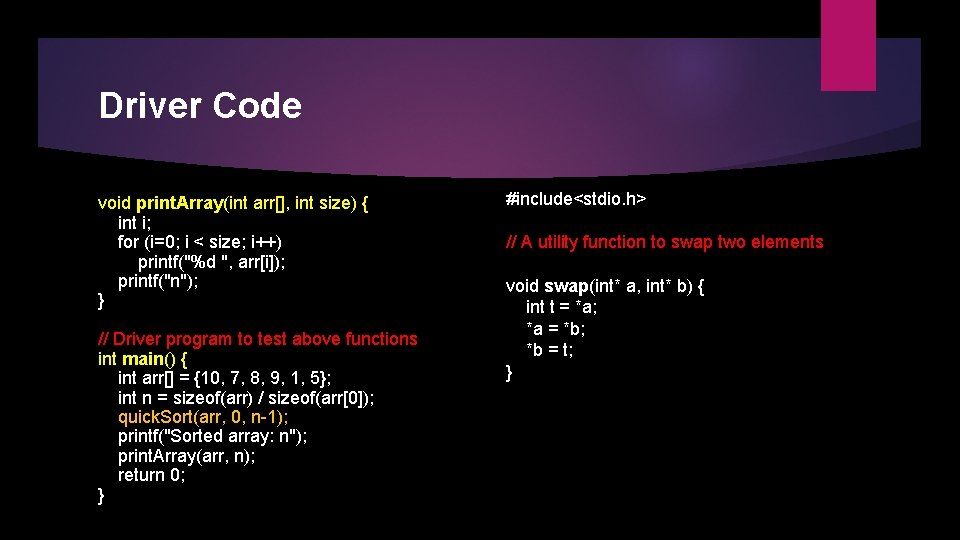Driver Code void print. Array(int arr[], int size) { int i; for (i=0; i < size; i++) printf("%d ", arr[i]); printf("n"); } // Driver program to test above functions int main() { int arr[] = {10, 7, 8, 9, 1, 5}; int n = sizeof(arr) / sizeof(arr); quick. Sort(arr, 0, n-1); printf("Sorted array: n"); print. Array(arr, n); return 0; } #include<stdio. h> // A utility function to swap two elements void swap(int* a, int* b) { int t = *a; *a = *b; *b = t; }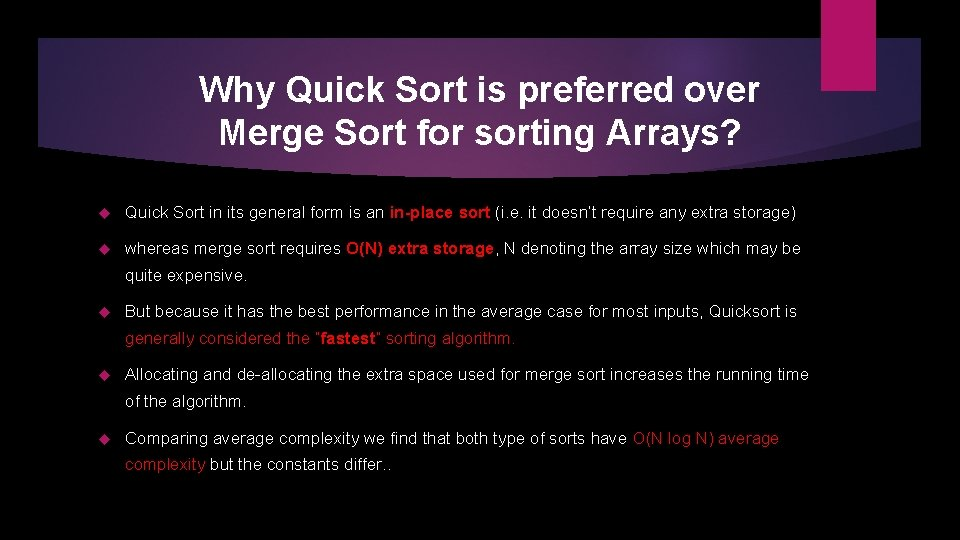Why Quick Sort is preferred over Merge Sort for sorting Arrays? Quick Sort in its general form is an in-place sort (i. e. it doesn’t require any extra storage) whereas merge sort requires O(N) extra storage, N denoting the array size which may be quite expensive. But because it has the best performance in the average case for most inputs, Quicksort is generally considered the “fastest” sorting algorithm. Allocating and de-allocating the extra space used for merge sort increases the running time of the algorithm. Comparing average complexity we find that both type of sorts have O(N log N) average complexity but the constants differ. .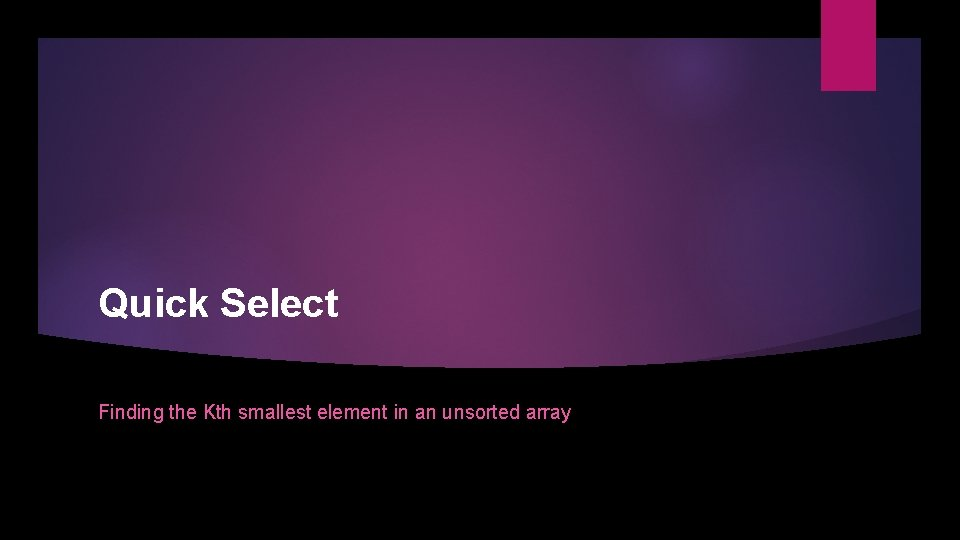Quick Select Finding the Kth smallest element in an unsorted arrayQuick Select Visualization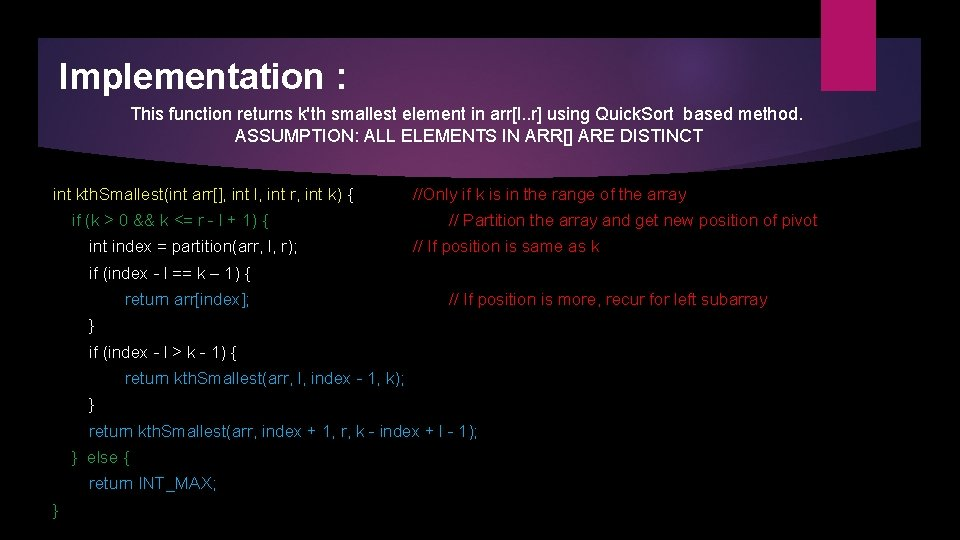Implementation : This function returns k'th smallest element in arr[l. . r] using Quick. Sort based method. ASSUMPTION: ALL ELEMENTS IN ARR[] ARE DISTINCT int kth. Smallest(int arr[], int l, int r, int k) { if (k > 0 && k <= r - l + 1) { int index = partition(arr, l, r); //Only if k is in the range of the array // Partition the array and get new position of pivot // If position is same as k if (index - l == k – 1) { return arr[index]; // If position is more, recur for left subarray } if (index - l > k - 1) { return kth. Smallest(arr, l, index - 1, k); } return kth. Smallest(arr, index + 1, r, k - index + l - 1); } else { return INT_MAX; }